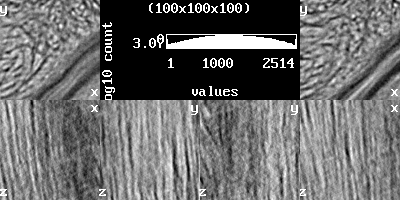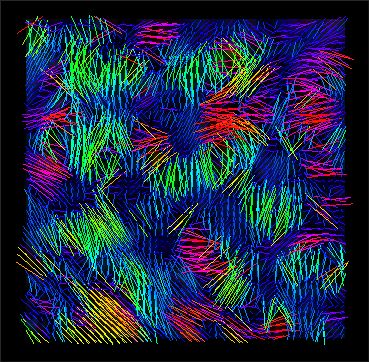## Connection (ImageXd)

Connection allows the mapping of fibres, planes, or spherical structures. It creates a tensor field based on all voxels and their surroundings.
For a possible visualization of your tensor data, I recommend the following paper: Leemans (2010) Visualization of Diffusion MRI Data
Additional software tools are: Slicer, OpenWalnut, MedInria, MRIcro, Trackvis, …

In this example, we use a part of an image stack from Julian Sartori (Thanks for the support!), which shows the achilles tendon of a rat.

To convert Dicom to the Nifti (nii) file format you can use dcm2niigui from Chris Rorden’s Neuropsychology Lab or 3DSlicer an open source software platform for medical image informatics, image processing, and three-dimensional visualization.

First step
Load an image stack and show its content.

▸ ImageXd

``` scalar.load stack.nii.gz scalar.info stack.nii.gz stack-info.txt scalar.analysis.image stack-analysis.png // more under the topic 'Histogram' scalar.setrange scalar.setcrop scalar.gradient stack-gradient.png // more under the topic 'View' ```scalar.analysisscalar.gradient

Second step
Calculate the tensor field.

▸ ImageXd

``` scalar.use scalar scalar.load "stack.nii.gz" thread.max := 6 scalar.connection 16 //scalar.connection.x2 10 // tensor.center tensor.save "stack_tensor.nii.gz" ```

▸ More details

``` // scalar.fullscan true // true(default) = scan only full spheres (without zero), false = scan incomplete spheres // scalar.connection.method 4 // 1=hdti 2=dti 3=ddti 4=hardhardi 5=softhardi(default) 6=hardi // algorithm for scalar.connection // hdti = similar to three direction (x,y,z)/2 diffusion imaging // dti = similar to seven direction (x,y,z + octants)/2 diffusion imaging // ddti = similar to thirteen direction 2*(x,y,z + octants + between x,y,z)/2 diffusion imaging // hardhardi = similar to high angular resolution diffusion imaging with limit of 64 orientation vectors // softhardi = similar to high angular resolution diffusion imaging with limit of 128 orientation vectors // hardi = similar to high angular resolution diffusion imaging // scalar.connection 16 // calculate a tensor field from scalar with voxel scan length // scalar.connection.x2 8 // calculate a half-sized tensor field from scalar with voxel scan length // scalar.connection.aniso 16 // calculate a tensor field from scalar with min (anisotropic) voxel scan length (only for hardi) // scalar.connection.aniso.x2 8 // calculate a half-sized tensor field from scalar with min (anisotropic) voxel scan length (only for hardi) ```

Third step
Evaluation (with 3DSlicer).stack_tensor.nii.gz (x,y view)stack_tensor.nii.gz (y,z view)stack_tensor.nii.gz (x,z view)